### Understanding Elementary Shapes-Solutions Ex-5.1

CBSE Class –VI Mathematics
NCERT Solutions
Chapter 5 Understanding Elementary Shapes (Ex. 5.1)

Question 1. What is the disadvantage in comparing line segments by mere observation?
Answer: There may be a chance of error due to improper viewing.
Question 2. Why is it better to use a divider than a ruler while measuring the length of a line segment?
Answer: It is better to use a divider than a ruler, because the thickness of the ruler may cause difficulties in reading off her length. However, divider gives up the accurate measurement.
Question 3.Draw any line segment, say $\overline{\text{AB}}.$ Take any point C lying in between A and B. Measure the lengths of AB, BC and AC. Is AB = AC + CB?
[Note: If A, B, C are any three points on a line, such that AC + CB = AB, hence we can be sure that C lies between A and B.]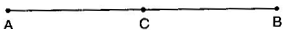AB = 6.5 cm, AC = 3cm, CB = 3.5 cm
AC + CB = 3 cm + 3.5 cm = 6.5 cm = AB
Question 4.If A, B, C are three points on a line such that AB = 5 cm, BC = 3cm and AC = 8 cm, which one of them lies between the other two?
Answer: $\overline{\text{AC}}$ is the longest line segment, thus B is the point between A and C.
Question 5.Verify whether D is the mid-point of $\overline{\text{AG}}.$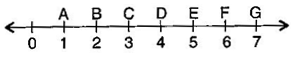Thus, D is the mid-point.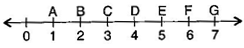Question 6. If B is the mid-point of $\overline{\text{AC}}$ and C is the mid-point of $\overline{\text{BD}},$ where A, B, C, D lie on a straight line, say why AB = CD?
Answer: B is the mid-point of $\overline{\text{AC}}$.
$\therefore$ AB = BC ……….(i)
And C is the mid-point of $\overline{\text{BD}}$.
$\therefore$ BC = CD ……….(ii)
From eq. (i) and (ii),
AB = CD
Question 7.Draw five triangles and measure their sides. Check in each case, of the sum of the lengths of any two sides is always less than the third side.
Answer: Yes, the sum of two sides of a triangle is always greater than the third side.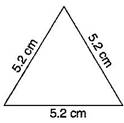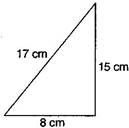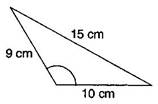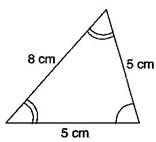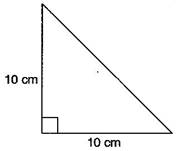apply Pythagorean theorem to find the hypotenuse i.e 14.14cm